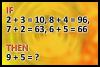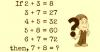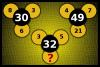BRAIN TEASERSBrain Teasers User Profile

# Anh Phạm

rank
357
points
7
See full ranking list
short ranking list
 355 bipinraj kunwar 7 356 Alexandra Santos 7 357 Anh Phạm 7 358 Murapala Sravani 7 359 mxeniak 7
 What is 9 + 5? If 2+3=10, 8+4=96, 7+2=63, 6+5=66 then 9+5=?Solve Math Puzzle If 2 + 3 = 8, 3 + 7 = 27, 4 + 5 = 32, 5 + 8 = 60, 6 + 7 = 72, then, 7 + 8 = ?Which number should replace the question mark? Which number should replace the question mark?